﻿ Chemical Composition Effects on Enthalpy Uncertainty in Natural Gas Energy Measurement System Using Orifice Meter in a Non-adiabatic Condition

### Chemical Composition Effects on Enthalpy Uncertainty in Natural Gas Energy Measurement System Using ...

Gunawan Marto, Harijono. A. Tjokronegoro, Edi Leksono, Nugraha, Ghufron ZaidOPEN ACCESSPEER-REVIEWED

## Chemical Composition Effects on Enthalpy Uncertainty in Natural Gas Energy Measurement System Using Orifice Meter in a Non-adiabatic Condition

Gunawan Marto1,, Harijono. A. Tjokronegoro1, Edi Leksono1, Nugraha1, Ghufron Zaid2

1Research Group of Engineering Physic Institut Teknologi Bandung, Jalan Ganesha 10 Bandung 40132, Indonesia

2Research Centre for Calibration, Instumentation dan Metrology (Pusli KIM-LIPI), Indonesia

### Abstract

This paper explainsan enthalpy uncertainty ofnatural gas energy measurement (custody transfer) using orifice meter in non adiabatic condition. The method of uncertainty analysis used in this paper was developed based on theuncertainty analysis of natural gas flow measurement using orifice meter at adiabatic condition based on AGA 3, 1992 (which reference by No 10.). In addition of non adiabatic condition includes: critical pressure, critical temperature, realtime pressure, realtime temperature and generalized correlation constanta. The measurement of enthalpy uncertaintyis referring to the Guide to the Eexpression of Uncertainty Measurementof the Guide in MetrologyWorking Group 1 of Joint Committee for Guide In Metrology, 2011. Based on the energy flow in orifice meter is 1000 Mmbtud, The combined uncertainty of f enthalpy is 3.86 x 10-7 Mmbtud (3.86 x 10-8 %) while the expandeduncertainty analysis results 7.73 x 10-7 Mmbtud (7.73 x 10-8 %)with confidence level 95%. This number of uncertaintyis smaller than the Measurement Permissive Error specified by legal metrology organization 0.1667%.

### At a glance: Figures

1
Prev Next

• Marto, Gunawan, et al. "Chemical Composition Effects on Enthalpy Uncertainty in Natural Gas Energy Measurement System Using Orifice Meter in a Non-adiabatic Condition." International Journal of Physics 2.5 (2014): 151-157.
• Marto, G. , Tjokronegoro, H. A. , Leksono, E. , Nugraha. , & Zaid, G. (2014). Chemical Composition Effects on Enthalpy Uncertainty in Natural Gas Energy Measurement System Using Orifice Meter in a Non-adiabatic Condition. International Journal of Physics, 2(5), 151-157.
• Marto, Gunawan, Harijono. A. Tjokronegoro, Edi Leksono, Nugraha, and Ghufron Zaid. "Chemical Composition Effects on Enthalpy Uncertainty in Natural Gas Energy Measurement System Using Orifice Meter in a Non-adiabatic Condition." International Journal of Physics 2, no. 5 (2014): 151-157.

 Import into BibTeX Import into EndNote Import into RefMan Import into RefWorks

### 1. Introduction

Precision and accuracy of a natural gas measurement using orifice meter are greatly determined by the collection of the measurement uncertainty comes from all of the involved measuring instruments. Uncertainty of the orifice meter is the uncertainty of real flow rate which is caused by the actual condition of the physical property of measured fluid, and the accuracy of installation parameters such as orifice diameter and beta ratio .

Orifice meters used in trade is necessary to have an ability to verify the measurement results. When choosing the meter, thoroughness and accuracy of the meter should be considered at first. If it’s going to be used in control applications, the inaccuracy should be within ± 2% acceptable range. If it's going to be used in operational applications, the inaccuracy should be within ± 5% acceptable range. If it's going to be used in operational applications, the inaccuracy should be within ± 0.5 - 2% acceptable range [2, 3].

According to AGA 3 1992, the uncertainty of flow rate is calculated by considering the discharge coefficient (Cd), orifice meter diameter (d) calculated based on flowing temperature (Tf), differential pressure (ΔP), velocity (Ev), dimension conversion constant (gc), universal constant (π), fluid density in flowing temperature (Pf, Tf), and expansion factor. The calculated expanded uncertainty of natural gas flow rate with 95% with expansion uncertainty value (U 95 S)2 from discharge coefficient (Cd) : 0.1936, expansion factor (Y) : 0.0009, orifice diameter (d) : 0.0114, pipe diameter (D): 0.011, pressure drop (ΔP): 0.0625, compressibility factor (Z): 0.0025, flowing temperature (Tf): 0.0156, and relative density (G) : 0.09 is ± 0.67% [1, 4].

To obtain the enthalpy uncertainty one has to have information aboutflow rate, heating value per volume unit, and relative density of natural gas values. The heating value is significantly determined by the measured composition of natural gas. The analysis of gas chromatography, heating value (Hv) calculation, and relative density calculation use reference of ASTM D 1945-96 (GPA 2261-95) and ASTM D 3588-98 (GPA 2172-96). Base on reference, results in heating value uncertainty of ± 0.25%, and relative density uncertainty of 0.003 .

National Bureau of Standards  (NBS) of France has done a research on a comparison between two methods of calculation of uncertainty of natural gas density. Natural gas using in research are pure methane and Liquefied Natural Gas (LNG). LNG is a mixture of methane, ethane, propane, and nitrogen. The methods are: (1) absolute densitometer based on the Archimedes principle; (2) correlation method based on measurement of composition and density. By the methods, the measurement results resulted in density uncertainty of pure methane of 0.02% and LNG of 0.025% .

Colorado Experimental Engineering Station Incorporated  (CESSI) has done a research on natural gas flow rate using 9 turbine meters. The standard of flow rate in CESSI facility is traceable to NIST. Capacity of each turbine is 0.7 m3/s to 10.7 m3/s. Operating pressure is 7174 kPa and operating temperature is ambient temperature. The research resulted uncertainty of flow rate is 0.28% to 0.30% dependent on natural gas flow rate .

Gunawan et al, 2012 , developed a calculation system of natural gas total energy measured using an orifice meter in non-adiabatic condition. In this measurement, besides volumetric and heating value parameters were considered, the net rate of generation such as heat loss caused by friction factor and also radiation heat from the sun were also taken into consideration .

In this uncertainty calculation of natural gas energy, the fluid that used is natural gas. The orifice meter measuring instrument system was operated in pressure between 29 to 30 bars. The operational temperature is adjusted between 25°C to 40°C. The compressibility factor variation is 0.85 to 1 .

Furthermore, this paper will discuss the empirical equation of sensitivity coefficient in section 2, the enthalpy uncertainty empirical equation section 3, the research methodology section 4, the calculation result and its discussion in section 5. The conclusion and acknowledgements will be delivered in closing chapter.

### 2. Empirical Equation of the Sensitivy Coeffiecient of Enthalpy

2.1. Empirical Equation of the Sensitivity Coefficient of Enthalpy

Uncertainty of energy measurement calculation is a basic and important foundation in natural gas measurement system using orifice meter, especially in custody transfer. Without information of uncertainty, measurement quality, modelling, and testing method can’t be quantified, untraceable, and the measurement result can’t be proven. Without estimation of uncertainty and its traceability to The International System of Units (SI) or other international standards, the measurement result is incomplete and meaningless [1, 2, 9].

In estimating the uncertainty value of total energy measurement using orifice meter, the orifice meter installation facility is deeply concerned and some variables need to be considered. Mathematical context between variables determines the sensitivity of measured amount of respective variables, each variable included in the energy balance equation has its own specific sensitivity coefficient. The derived coefficient in general wasis based on mathematical equation or approximated from curve or table calculation .

One dimensional equation of total energy in orifice meter measuring system is a function of enthalpy value (H), kinetic energy as flow rate function (v), height (z), net rate of generation (XG), and work produced by orifice meter system (W). This relationship is expressed mathematically as [10, 11, 12]: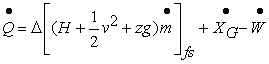(1)

Where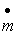is mass flow rate,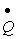is total energy,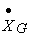is nate rate of generation and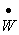is work. Equation (1) shows total enthalpy calculation is a sum of sensible enthalpy value and real enthalpy value. The sensible enthalpy is calculated based on hrat capacity in ideal condition, while rhe real enthalpy such as the compressibility factor function (Z) is calculated according to Generalized Correlation as follows [10, 11, 12].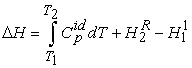(2)

Equation (2) explains the correlation between total enthalpy (ΔH), a sum of sensible enthalpy value calculated based on heat capacity value in ideal condition, and real enthalpy value (HR). To calculate the sensitivity value from the sum of enthalpy value, the equation of the total enthalpy―consisted of ideal enthalpy and real enthalpy should be derived (first derivative). Ideal enthalpy value is expressed as [10, 11, 12]: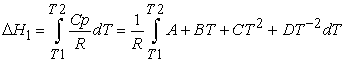(3)

Equation (3) is a non-linear equation: a heat capacity function dependent on temperature. The solution of equation (3) is given by [10, 11, 12]: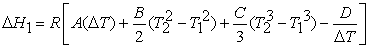(4)

Where ΔT is the difference between T2 and T1. Equation (4) explains the ideal enthalpy value as the product of ideal gas constant (R) expressed in (J mol-1 K-1) and the heat capacity derived from empirical equation of natural gas component constants (A, B, C, D) as a temperature function. In equation (5), if the temperature of upstream part (T1) is almost the same as the temperature of downstream part (T2), then ΔH1 is assumed to be significantly influenced by the value of ideal gas constant (R), gas component constant (A), and temperature difference between upstream and downstream (ΔT).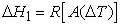(5)

The sensitivity coefficient of ideal enthalpy is the first derivative of ideal enthalpy and expressed as: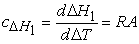(6)

Equation (6) explains the sensitivity value of ideal enthalpy as the first derivative of ideal enthalpy value. This coefficient of sensitivity value is the product of ideal gas constant (R) and natural gas component constant (A). The total value of real enthalpy is expressed as: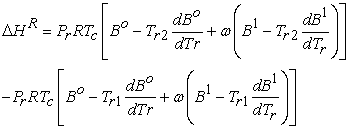(7)

Equation (7) explains the real enthalpy value. The real enthalpy value is significantly influenced by the value of real pressure (Tr), ideal gas constant (R), critical temperature (Tc), and generalized correlation constant as a temperature function (Bo,B1,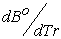, and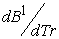).

The value of pressure and operatioanl temperatire is given by: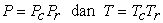(8)

where Pc is critical Pressure, Pr real Pressure, Tc is critical temperature and Tr is real temperature. To calculate the coefficient value of real enthalpy, the first derivative of real enthalpy with respective to the each building blocks of the real enthalpy function components should be obtained. For example, the sensitivity coefficient of real pressure is a partial derivative of real enthalpy (HR) respective to real pressure (Pr). This equation is expressed as: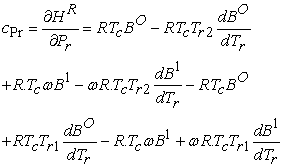(9)

Equation (9) explains the sensitivity coefficient of real enthalpy respective to the real pressure as a function of real pressure (Tr), ideal gas constant (R), critical temperature (Tc), and generalized correlation constant as temperature function (Bo,B1,, and).

By using the same method, the sensitivity coefficient of other components/variables of the real enthalpy function is given by Table 1.

#### Table 1. Sensitivity Coefficient of Real Enthalpy (HR)

2.2. Empirical Equation of Enthalpy Uncertainty

Uncertainty of total energy that is obtained by orifice meter is considerably influencing the measurement result. For example, in determining the enthalpy uncertainty (H), enthalpy value is a function of heat capacity as temperature function (T). Based on this fact, the enthalpy uncertainty is estimated from the temperature uncertainty. This is different from the determination of kinetic energy uncertainty. The kinetic energy uncertainty calculation result is determined by the fluid flow rate, therefore it is estimated from the uncertainty of flow rate [1, 13].

The occurrence of error in every measurement is a basic in determining uncertainty. Error is the difference between measurement result and the true value. With the presence of error, a measurement result could have deviated from its true value. Because error can’t be solved with correction and eliminated due to human limitations or measuring instruments used in measuring system, a lot of mathematical theories are used to anticipate this limitation. Probability theory is used in measurement in order to handle the irremovable error .

Determination of error is also related to the standard deviation with probability. The measurement results can also be related to probability. Due to the presence of doubts from various factors in a measurement, these doubts can be addressed with the determined-distribution measurement probability. The combination of various distributions will result in a normal distribution described in Central Limit Theorem. The standard deviation of this normal distribution will be used as a basis in determining the measurement of uncertainty .

By taking a lot of things and references in determining the value of uncertainty into account, the determination of uncertainty value of each function of orifice meter total energy measurement can be done empirically or even analytically.

After obtaining the uncertainty value of each component from orifice meter energy equation function, the calculation of combined uncertainty with estimated error from Taylor Series is performed. It results in combined uncertainty value of :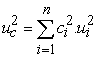(13)

Where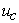is combined uncertainty,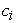is sensitivity coefficient of entalphy component and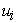is uncertainty of entalphy component. Equation (13) explains square root of combined uncertainty. Combined uncertainty can therefore be viewed as a sum of the product of square sensitivity coefficient of entalphy component and square uncertainty of entalphy component .

The uc symbol is obtained from the abbreviation of combined uncertainty. To get a range with a certain probability, the combined uncertainty is multiplied by a constant called the coverage factor. In general, the 95% confidence level is used for reporting measurement uncertainty. If the normal distribution obtained from the coverage factor is given the k symbol, it will give k=2 .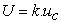(14)

Equation (14) explains the expanded uncertainty (U), which is a product of combined uncertainty (uc) and coverage factor (k) in order to obtain minimal probability from measurement result.

### 3. Calculation Methodology of Uncertainty

3.1. Materials

The natural gas used in this uncertainty calculation is a mixture of natural gas from various locations with up to 21 types of components. The largest content of this natural gas is methane gas (82.54% mole). This natural gas also contains a heavy fraction of propane (C3H8), butane (C4H10), pentane (C5H12), and hexane (C6H14). Table 2 shows the results of laboratory analysis of the composition of the natural gas used in the calculation of the total uncertainty of energy in orifice meter .

#### Table 2. Laboratory Analysis of Natural Gas Composition

3.2. Instrumentation

The instrumentation system used in this research is depicted by Figure 1. The system constitudes (1) Temperature -1 (2) Gas Analyzer (3) Pressure gauge-1 (4) Flow Computer Non Adiabatic (5) Temperature Transmitter-2 (6) Differential Pressure (7) Pressure Transmitter-2 (8) Flow Computer Adiabatic and (9) Meter orifice installation .

The data needed for calculation of uncertaintyis operational conditionsis given by Table 3.

3.3. Procedure

The methodology used in this research follows the procedure from Joint Committee for Guide in Metrology: Guide the expression of uncertainty measurement procedure can be explained as described below:

a. Retrieval of dimension data or orifice meter size in one of the calibration laboratories in charge of metrology activities.

b. Data collection of operating conditions such as temperature, pressure, gas composition and other operating data in the orifice meter installation take place in one of the Oil and Gas Company.

c. Perform sensitivity coefficients mathematical modeling of each component function from energy equation.

d. Perform uncertainty calculations both analytical and empirical of each component function from energy balance equation based on the certificate, calibration results and orifice meter installation operating conditions.

e. Perform combined and stretch uncertainty calculation which is the multiplication of the combined uncertainty with the coverage factor (k) and confidence level of 95%.

f. Conduct an analysis of stretch uncertainty value compared with the technical requirements that have been issued by organizations in charge of traceability and standards.

### 4. Calculation Results and Discusion

4.1. Sensitivity Coefficient and Combined Enthalpy Uncertainty (uc)

Referring data from Table 2 and Table 3 are used to calculate the sensitivity coefficient value and the uncertainty value of the function component from the energy equation

The sensitivity coefficient of enthalpy equation shown in equation (7) is a function of the ideal gas constant (R) and the constant of a natural gas component (A). Because of the enthalpy uncertainty is substantially influenced by temperature (T).Therefore, the value of enthalpy uncertainty is taken from the temperature uncertainty (uT)that obtained froma calibration sertificate with value of 0.0012. By using R = 3.814 J/mol.K, the sensitivity coefficient and enthalpy uncertainty are shown below (Table 4).

#### Table4. Calculation of Ideal Enthalpy Uncertainty

By using the same method, the sensitivity coefficient and combined uncertainty is shown in Table 5. From Table 5, it can be deduced that the total combined uncertainty of enthalpy is influenced by the factors: uncertainty of ideal enthalpy; uncertainty of real pressure; uncertainty of generalized correlation coefficient; uncertainty of critical temperature; and uncertainty of real temperature.

#### Table 5. Calculation of Total Uncertainty of Enthalpy (H) in Orifice Meter

From the tabel, we can see that the natural gas of B has the largest uncertainty (0.000489Joule/mole (8.59 x 10-7 Mmbtud)) and the natural gas of A has the smallest uncertainty (0.000396 Joule/mole (6.95 x 10-7Mmbtud)).Relation between the density and compressibility is explained on Figure 1. According to Figure 1(a), the natural gas of B has the smallest density of 23.285 kg/m3and the largest compressibility factor of 0.938.The natural gas composition of A has the largest density of 27.245 kg/m3 and the smallest compressibility factor of 0.9114. The Natural gas Bhasproperties close to the ideal gas.

Figure 2. (a) Density Relation to Compressibility. (b) Compressibility Relation and Expanded Uncertainty

Furthermore, according to Figure 2 (b), the natural gas with large density (Gas A)within a transportation process going across a piping system, when experiencing a pressure drop. Gas components with larger density (propane, butane, and gases with heavier molecular weight) will be condensed into a liquid whereas gases with lighter phase such as methane and ethane will pass through orifice meter. From Table 2, it can be explained that light phase gases passing the orifice meter. The natural gas composition of A has light phase of 89.96% and natural gas with B composition has a light phase of 94.85%. Both of the gases can be seen that if the amount of light phase gases passing the orifice meter is larger, its uncertainty value is also larger.

4.2. Accuracy and Precision

The calculation results for all kind of gas compositions show that the largest value of expanded uncertainty is the natural gas with B composition (8.59x10-7 Mmbtud (±8.59x108%)). This value is smaller than the calculation result from previous research that resulted of expanded uncertainty of enthalpy value based on adiabatic condition is ± 0.25 %. ( Paul J. La Nasa et al, 2002). This calculation result shows that the calculation of enthalpy uncertainty in a non-adiabatic condition give better precision and acuration.

According to the organization of legal metrology, the uncertainty value should be smaller than one-third (1/3) of the Maximum Permissible Error (MPE). If the magnitude of Maximum Permissible Error is ± 0.50 %, the magnitude of uncertainty shouldn’t be more than 0.1667%. The total uncertainty of enthalpy in a non-adiabatic condition is 8.59 x 10-7 Mmbtud (±8.59 x 10-8%), which means this value is still smaller than required (0.1667%). This also means that the energy measurement result in a non-adiabatic condition method is acceptable and can be proposed as a new method of calculating the total natural gas energy using orifice meter.

### 5. Conclusion

The calculation of total uncertainty of enthalpy in a non-adiabatic condition has been developed from the calculation method of natural gas flow rate uncertainty with orifice meter based on AGA 3, 1992  and Heating value (Hv) uncertainty calculation method. Total expanded uncertainty of enthalpy in this a non-adiabatic condition is calculated with 95% confidence level and coverage factor of 2 (k=2). This total expanded uncertainty of enthalpy is a sum of total combined uncertainty of ideal enthalpy and real enthalpy.

The calculation result of the highest total combined uncertainty of enthalpy (for B natural gas composition) is 3.86 x 10-7 Mmbtud (3.86 x 10-8 %) and the calculation result of expanded uncertainty is 7.73 x 10-7Mmbtud (7.73 x 10-8 %). The value of this expanded uncertainty is smaller than required (0.1667%) which means the method of energy measurement result in a non-adiabatic condition is acceptable and can be proposed as a new method of calculating the natural gas total energy using orifice meter.

### Acknowledgement

The authors express gratitude for the Centre of Metrological Human Resources Development and Directorate of Metrology of Ministry of Trade of Republic of Indonesia that provided assistance and facilities for data acquisition for metrological research in year of 2013/2014.

### References

  AGA3. “Orifice Metering Of Natural Gas And Other Related Hydrocarbon Fluids3:Natural Gas Applications”, American Gas Association, Third Edition, August 1992.In article  Upp, E loy “Fluid Measurement A Practical Guide to Accurate Flow Measurement” (2nd en). USA. Gulf Professional Publishing, 2002.In article  Yanuar YAP. “The Analysis of Gas Meter Uncertainty Using Orifice Flowmeter and Ultrasonic Flowmeter”. Chemical Engineering Department, The University of Indonesia, 2012.In article  Paul J. La Nasa. “Overall Measurement Accuracy. “CPL & Associates, P.O. Box 801304, Houston, TX 77280-1304, 2003.In article  M. Roncier, R. Philippe, J. Saint-Just, and F. Dewerdt, Estimated Uncertainty of Calculated Liquefied Natural Gas Density from a Comparison of NBS and Gaz de France Densimeter Test Facilities, Journal Of Research of the National Bureau of Standards, Vol. 88, No. 3, May-June 1983.In article CrossRef  Aaron Jhonson, Tom kegel, Uncertainity and Traceability for CESSI lowa Natural Gas Facilities, Jounal of Research of National Institut of Standard and Technology, volume 109, Number 3, May-june, 2004.In article  Gunawan, Harijono A Tjokronegoro, Edi Leksono, Nugraha “ The effect of non adibatic condition to pressure drop and volumetric rate of natural gas measurment using orifice flowmeter “ National Seminar of Calibration, instrumentation and metrology, Research Centre For Calibration, Instumentation dan Metrology (Pusli KIM-LIPI), Indonesia, 39th, 2013.In article  Mahmood Farzaneh-Gord, Azad Khamforoush, ShahramHashemi and HosseinPourkhademNamin,“Computing Thermal Properties of Natural Gasby Utilizing AGA8 Equation of State”International Journal of Chemical Engineering and Applications, Vol. 1, No. 1, June 2010, page No.20-24.In article  Ibrahim Reda “Method To Calculation Uncertainties In Measuring Shortwave Solar Irradiation Using Thermopile and Semiconductor Solar Radiometer” National Renewable Energy Laboratory, Technical Report NREL/TP-3B10-52194, July, 2011.In article  Smith.J.M, Abbott.M.M, Van Ness.H.C. “Introduction to Chemical Engineering Thermodynamic“ Fifth edition, The Mcgraw Hill Companies, Inc, Singapore, 1996.In article  McCabe”Unit Operation Of Chemical Engineeering” fifth edition, McGraw Hill Chemical Engineering Series, 1993.In article  Bird.B.R, Steaward E.W, “Transport Phenomena” Second Edition, John Wiley and Sons, Inc, 2001.In article  JCGM/WG 1. “Guide the expression of uncertainty measurement”. Working Group 1 of Joint Committee for Guide In Metrology, 2011.In article  Kirkup, Les and Frenkel, Bob. “An Introduction to Uncertainty in Measurement”. New York, Cambridge, 2006In article CrossRef  ISO (1993). ”Guide to the Expression of Uncertainty in Measurement”, Geneva, International Organisation for Standardisation (corrected and reprinted 1995).In article  James T Nakos. “Uncertainity Analysis of Steady State Incident Flux Measurements In Hydrocarbon Fuel Fires”. Sandia Report, Sandia National Laboratories, 2005.In article  Francisco J, “Uncertainty Analysis For Experimental Heat Transfer Data Obtained By Wilson Plot Methode”. Journal Of Thermal Science, Volume 17 No 2, pp 471-481, 2013.In article CrossRef  Gunawan, Harijono A Tjokronegoro, Edi Leksono, NUgraha “Effect of Heat loss Caused By Friction Factor To Natural Gas Measurement System By Orifice Meter “Jurnal of Instrumentasi, Research Centre For Calibration, Instumentation dan Metrology, 2012.In article  Gunawan, Harijono A Tjokronegoro, Edi Leksono, Nugraha “Effect Of Heat Radiation To Natural Gas Measurement System By Orifice Meter “ Jurnal of Indonesian Physical Society, 2014.In article